## Example Questions

2 Next →

### Example Question #11 : How To Find The Area Of A Parallelogram

A parallelogram has a base of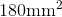and a height measurement that isthe base length. Find the area of the parallelogram.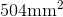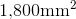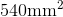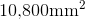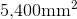Explanation:

By definition a parallelogram has two sets of opposite sides that are congruent/parallel. However, to find the area of a paralleogram, you need to know the base and height lengths. Since this problem provides both the base and height measurements, apply the formula: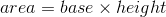Before applying the formula you must findof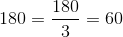The solution is: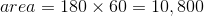Note: when working with multiples of ten remove zeros and then tack back onto the product.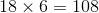There were two total zeros in the factors, so tack on two zeros to the product: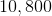### Example Question #12 : How To Find The Area Of A Parallelogram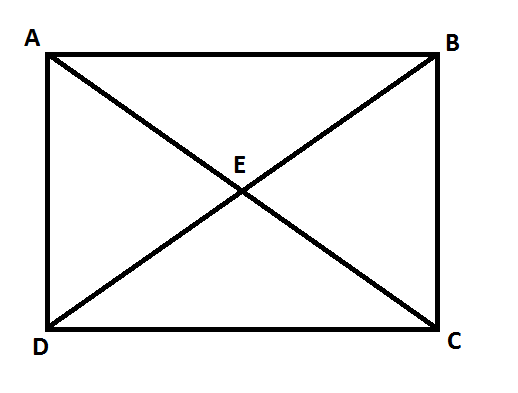ABCD is a rectangle.

Quantity A: The area of AEB

Quantity B: The area BEC

Quantity B is greater.

Quantity A is greater.

The two quantities are equal.

The relationship cannot be determined.

The two quantities are equal.

Explanation:

The area of a triangle is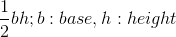Consider the rectangle ABCDAs a rectangle: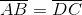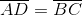With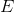appearing directly in the center of the rectangle.

The area of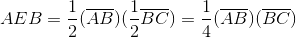Notice that the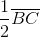term corresponds to the triangle's height.

The area of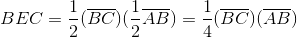The two quantities are equal.

### Example Question #13 : How To Find The Area Of A Parallelogram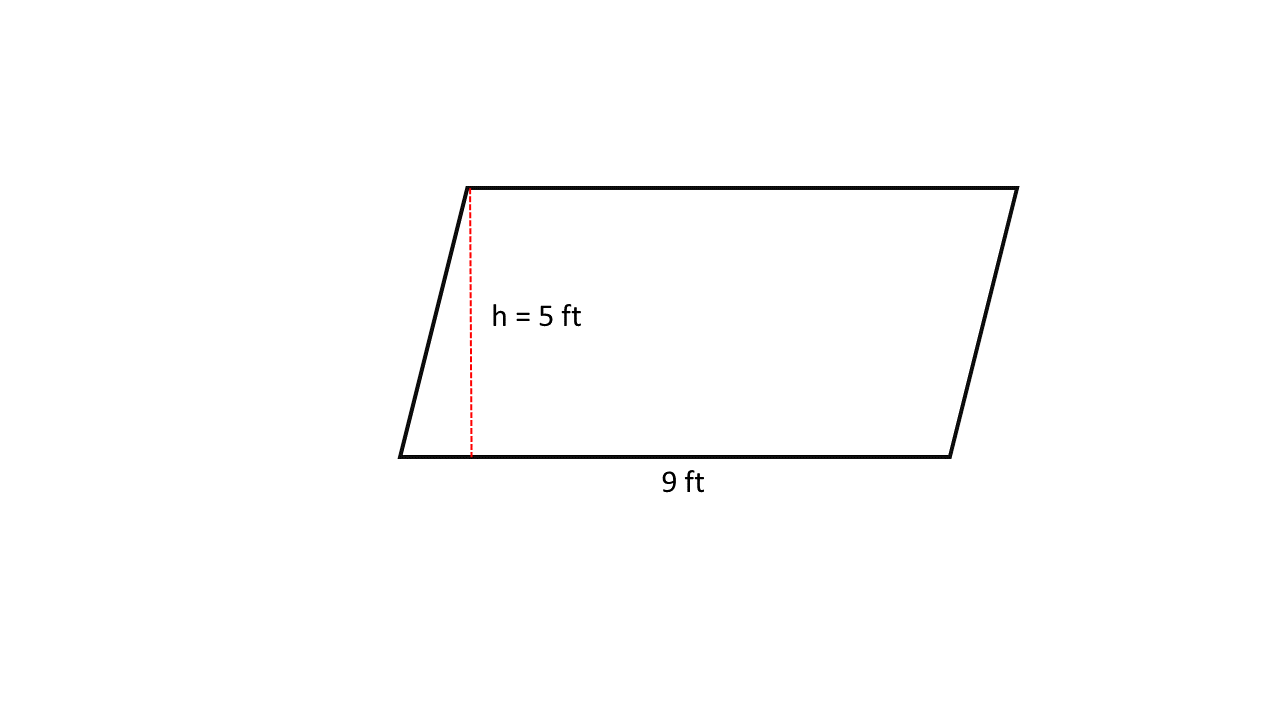Using the parallelogram shown above, find the area.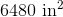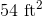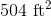Not enough information is provided.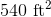Explanation:

This problem provides both the base and height measurements, thus apply the formula: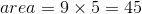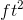To find an equivalent answer in inches, you must convert the measurements to inches FIRST, and then multiply: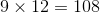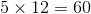Therefore, our area in square inches is: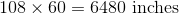2 Next →

### All GRE Math Resources